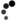# Encryption

Encrypt Decrypt
##### Implementation details

The AES-256 (CBC) encryption we apply here works as follows:

• calculate the MD5 hashes of the key and the Intialisation Vector
• determine the actual key and Initialisation Vector based on these hashes
• encrypt the given text with this key and Initialisation Vector with 256 bits AES in CBC (Cipher Block Chaining) mode, after which the result is encoded with Base64 encoding
• for decryption, the given text is decoded from Base64 encoding and decrypted with the above-mentioned key and Initialisation Vector with 256-bit AES in Cipher Block Chaining mode (CBC)

In case of encryption, the Initialisation Vector is pasted to the text before it is encrypted. Decryption eliminates the initialisation vector from the beginning of the decrypted text.

Note: spaces and the like at the beginning and end of the entry are not removed and are therefore important for successful decryption!

##### What is AES?

In cryptography, Advanced Encryption Standard (AES) is a computer encryption technology. It is the successor to the "Data Encryption Standard" (DES). AES is a subset of the Rijndael algorithm where the block size is 128-bit, and the key 128, 192 or 256 bits. Rijndael itself can all block sizes and keys that are a 32-bit multiplication with a minimum of 128-bit and a maximum of 256-bit.

Source: Wikipedia

##### How to program in PHP?
```<?php

define('CRYPT_CIPHER',  MCRYPT_RIJNDAEL_256);				// encryption algorithm
define('CRYPT_MODE',    MCRYPT_MODE_CBC);					// encryption algorithm mode

define('CRYPT_KEY', 'f6631c0e3de8bff772c9dcfed7353baa');	// encryption key

\$text = 'This is a text';									// plain text to encrypt

// determine initialization vector size and initialization vector
\$ivSize  = mcrypt_get_iv_size(CRYPT_CIPHER, CRYPT_MODE);
\$iv      = substr(CRYPT_IV, 0, \$ivSize);

// determine key size and key
\$keySize = mcrypt_get_key_size(CRYPT_CIPHER, CRYPT_MODE);
\$key     = substr(CRYPT_KEY, 0, \$keySize);

// encrypt text
\$result = mcrypt_encrypt(CRYPT_CIPHER, \$key, \$text, CRYPT_MODE, \$iv);

// result contains binary string, two major it anywhere convert
// it to optimized ascii using base64 encoding
\$result = base64_encode(\$result);

// \$result now contains string:
// 'BggvzMUWJ5Hp4g3KiGVnWjsjiJ+r97MXqE8ujbchHqI='```

Call now  +31207775488  if you need another tool!